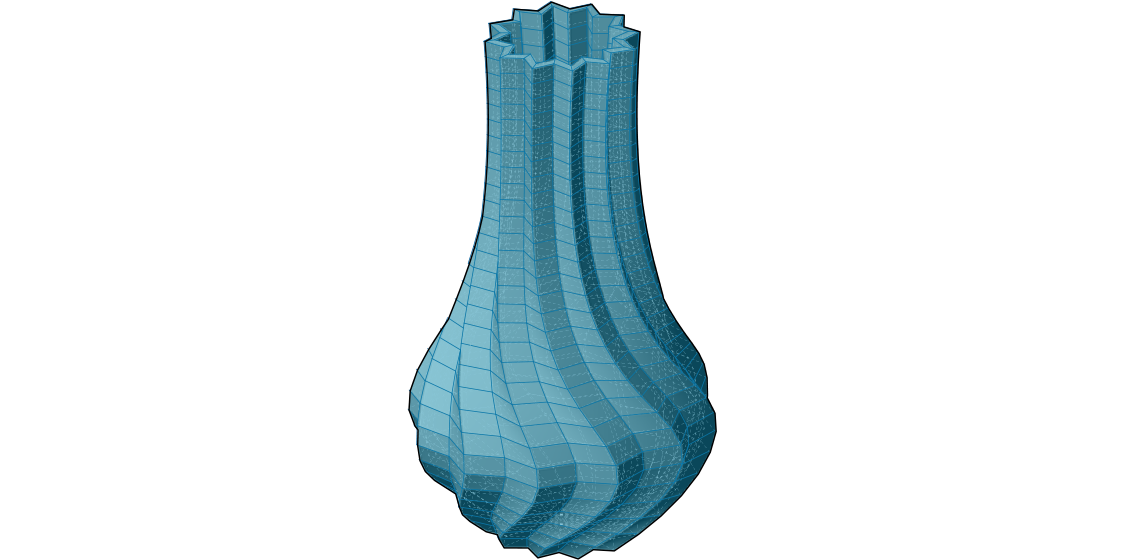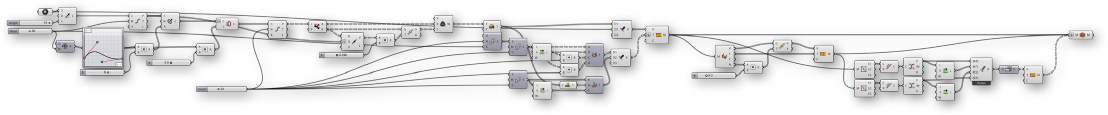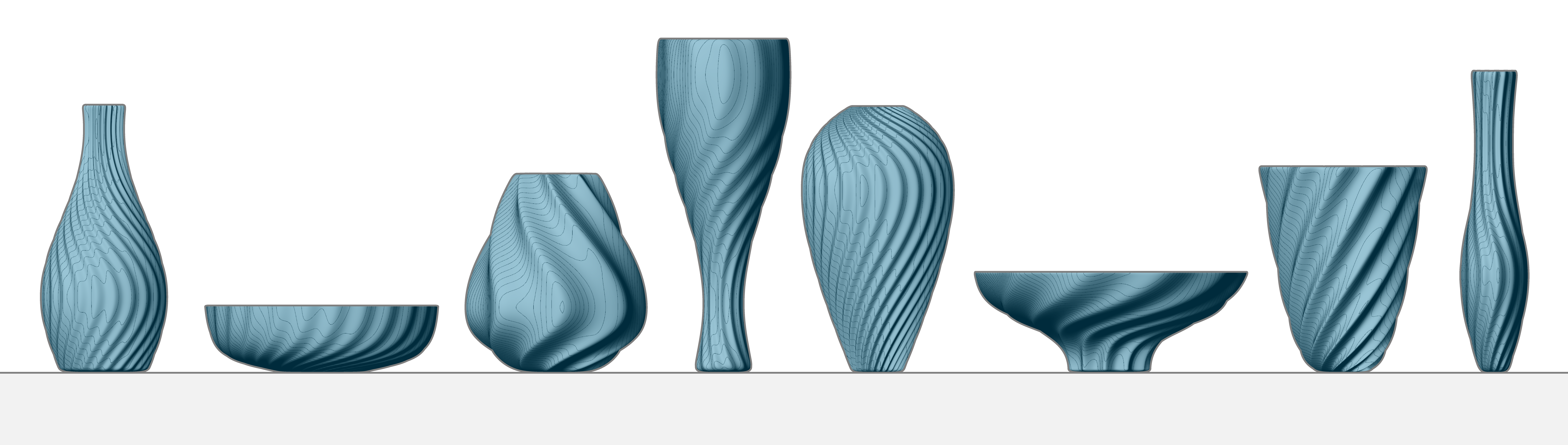### 1.6.6 Working with Mesh Geometry

##### In this section, we will work through an exercise file for producing a complete mesh solid. By the end of this exercise, we will have a dynamic definition to produce custom vases that can be 3D printed.

Since this definition is somewhat longer than previous examples in this primer, we will first walk through the basic steps we will take: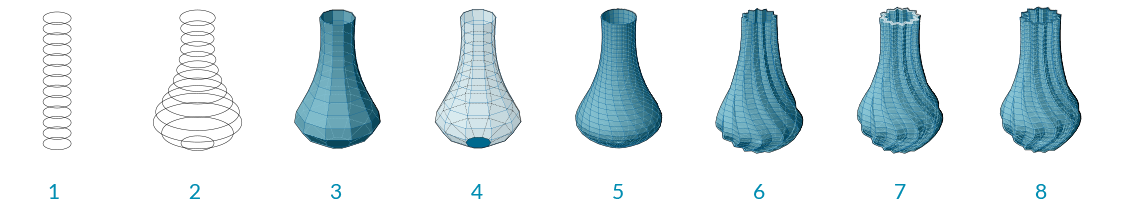1. Create a series of circles as a base cylinder
2. Use a Graph Mapper component to define the profile of our vase
3. Construct the topology of the mesh faces to produce a single mesh surface
4. Cap the bottom of the mesh
5. Introduce a twist to the vertical orientation for a more dynamic form
6. Add corrugated ridges for a textured vase
7. Offset the mesh surface to give the vase thickness
8. Cap the top gap between the two surfaces to produce a closed solid
01. Start a new definition, type Ctrl-N (in Grasshopper)
02. Params/Geometry/Point - Drag and drop a Point container onto the canvas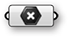03. Reference a point in Rhino by right-clicking the Point component and selecting "Set one point". This will serve as the origin point of our vase.

You can create a point manually in Grasshopper by double-clicking the canvas to bring up the search window, then typing the coordinates of the point separated by commas, such as: '0,0,0' (without quotes)
04. Params/Input/Number Slider - Drag and drop a Number Slider component onto the canvas and set the following values:
Name: Length
Lower Limit: 1
Upper Limit: 10
05. Curve/Primitive/Line SDL - Drag and drop a Line SDL component onto the canvas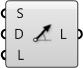06. Connect the Point component to the Start (S) input of the Line SDL component, and connect the Number Slider to the Length (L) input.

The default Direction (D) value of **Line SDL** is the Unit Z vector, which is what we will use for this example
07. Params/Input/Number Slider - Drag and drop a Number Slider onto the canvas and set the following values:
Name: V Count
Rounding: Integer
Lower Limit: 1
Upper Limit: 100
08. Curve/Division/Divide Curve - Drag and drop a Divide Curve component onto the canvas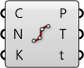09. Connect the Line (L) output of the Line SDL component to the Curve (C) input of the Divide Curve component
10. Connect the V Count number slider to the Count (N) input of the Divide Curve component
11. Curve/Primitive/Circle CNR - Drag and drop a Circle CNR component onto the canvas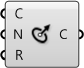12. Connect the Points (P) output of the Divide Curve component to the Center (C) input of the Circle CNR component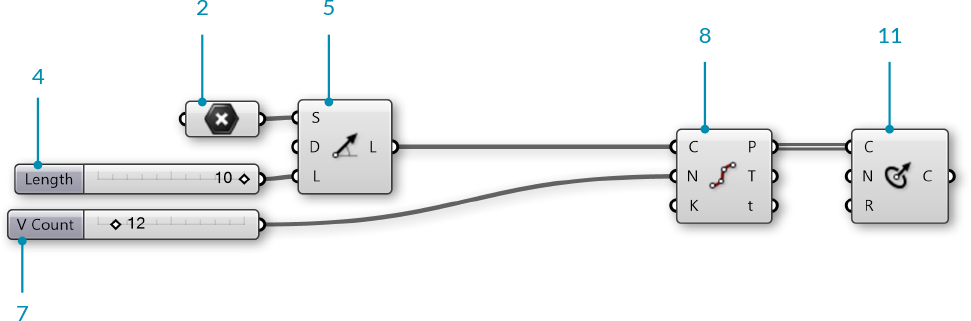We have a series of circles stacked vertically. We will use these to make the profile of our vase.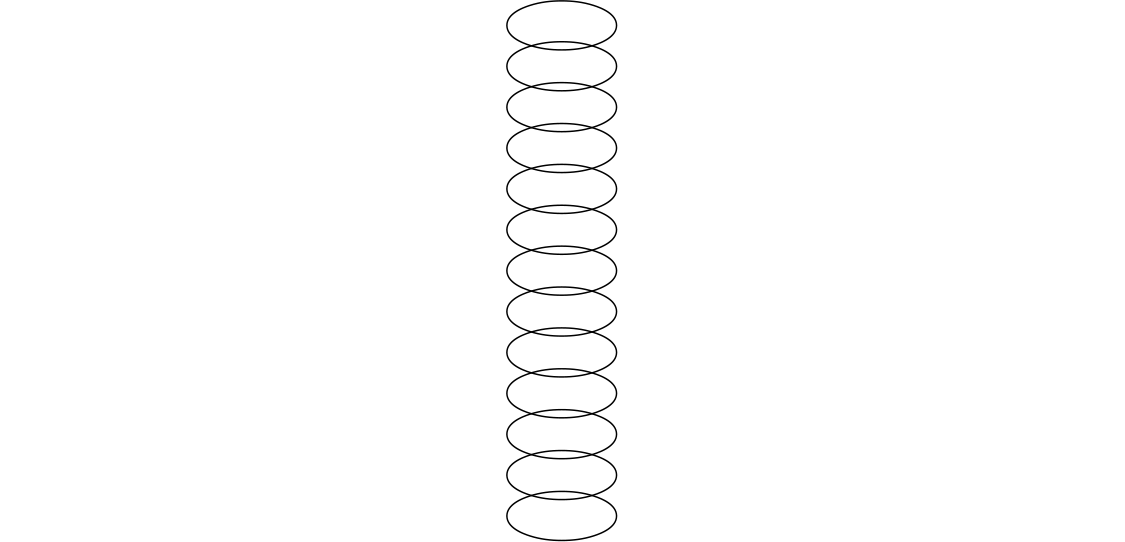Next, we will use a Graph Mapper to control the radii of the circles.

13. Sets/Sequence/Range - Drag and drop a Range component onto the canvas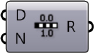14. Connect the V Count number slider to the Steps (N) input of the Range component
15. Params/Input/Graph Mapper - Drag and drop a Graph Mapper component onto the canvas
16. Right-click the Graph Mapper, click 'Graph Types' from the menu and select 'Bezier'
17. Params/Input/Number Slider - Drag and drop a Number Slider component onto the canvas and set the following values:
Name: Width
Lower Limit: 0
Upper Limit: 10
18. Maths/Operators/Multiplication - Drag and drop a Multiplication component onto the canvas19. Connect the Graph Mapper and the Width number slider to the A and B inputs of the Multiplication component
20. Connect the Result (R) output of the Multiplication component to the Radius (R) input of the Circle CNR component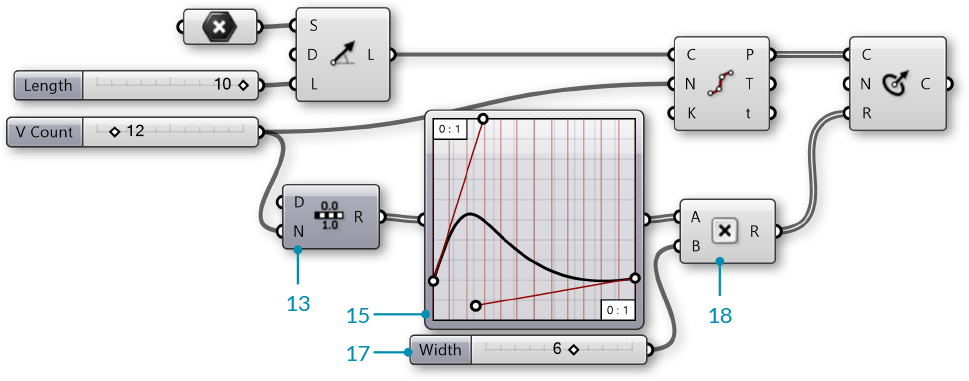Use the handles on the Graph Mapper to adjust the profile of the circles.

NOTE: It is important to make sure the start point of the bezier curve on the Graph Mapper is not at zero. By lifting the start point to a number greater than zero, we produce a flat base for our vase.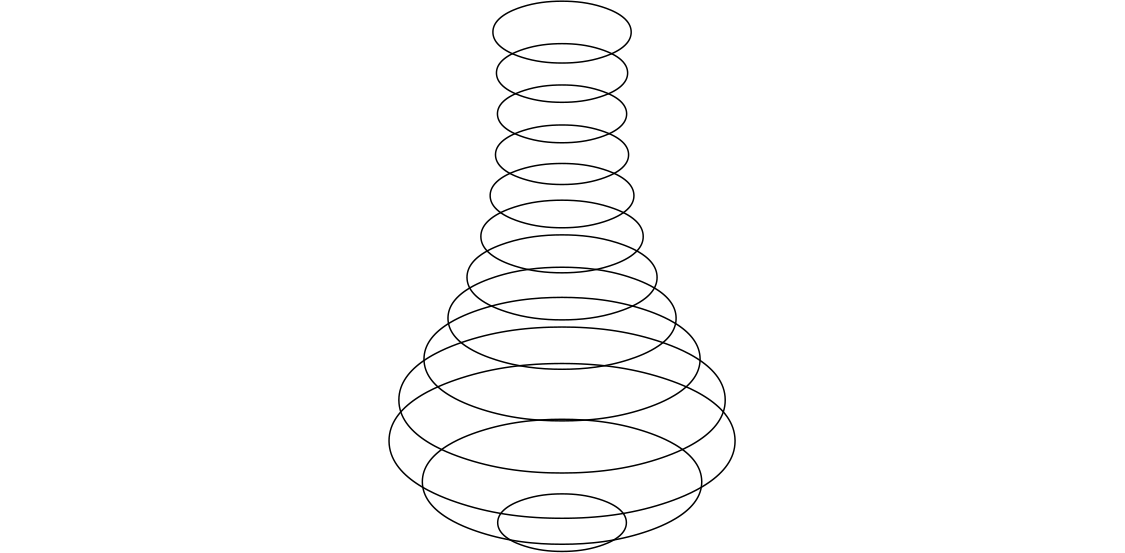We now have a profile for our vase. Next, we will construct a mesh surface. This will require creating mesh vertices and defining mesh faces according to the index of those vertices.

21. Params/Input/Number Slider - Drag and drop a Number Slider component onto the canvas and set the following values:
Name: U Count
Rounding: Even
Lower Limit: 2
Upper Limit: 100
22. Curve/Division/Divide Curve - Drag and drop a Divide Curve component onto the canvas
23. Connect the Circle (C) output of the Circle CNR component to the the Curve (C) input of the Divide Curve component, and connect the U Count number slider to the Count (N) input

The Points(P) output of this component are the vertices we will use for our mesh
24. Sets/Sequence/Series - Drag and drop two Series components onto the canvas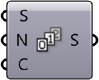25. Connect the U Count number slider to the Step (N) input of the first Series component, and connect the V Count number slider to the Count (C) input of the same Series component
26. Connect the Series (S) output of the first Series component to the Start (S) input of the second Series component, and connect the U Count number slider to the Count (C) input
27. Sets/List/Shift List - Drag and drop a Shift List component onto the canvas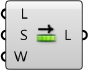28. Connect the output of the second Series component to the List (L) input of the Shift List component
29. Maths/Operators/Addition - Drag and drop two Addition components onto the canvas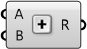30. Connect the output of the second Series component and the U Count number slider to the A and B inputs of the first Addition component
31. Connect the output of the Shift List component and the U Count number slider to the A and B inputs of the second Addition component
32. Mesh/Primitive/Mesh Quad - Drag and drop a Mesh Quad component onto the canvas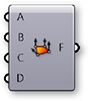33. Connect the following to the inputs of the Mesh Quad component:
A - Second **Series** component
B - **Shift List**

We have just create the initial topology for our mesh. These faces will be combined with the vertices. The order of these connections is crucial, so go ahead and double check all the connections at this point!
34. Sets/Tree/Flatten - Drag and drop a Flatten Tree component onto tha canvas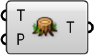35. Connect the Points (P) output of the Divide Curve component to the Tree (T) input of the Flatten Tree Component
36. Mesh/Primitive/Construct Mesh - Drag and drop a Construct Mesh component onto the canvas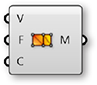37. Connect the Tree (T) output of the Flatten Tree component to the Vertices (V) input of the Construct Mesh component
38. Connect the Face (F) output of the Mesh Quad component to the Faces (F) input of the Construct Mesh component. Right-click the F (Faces) input and select 'Flatten'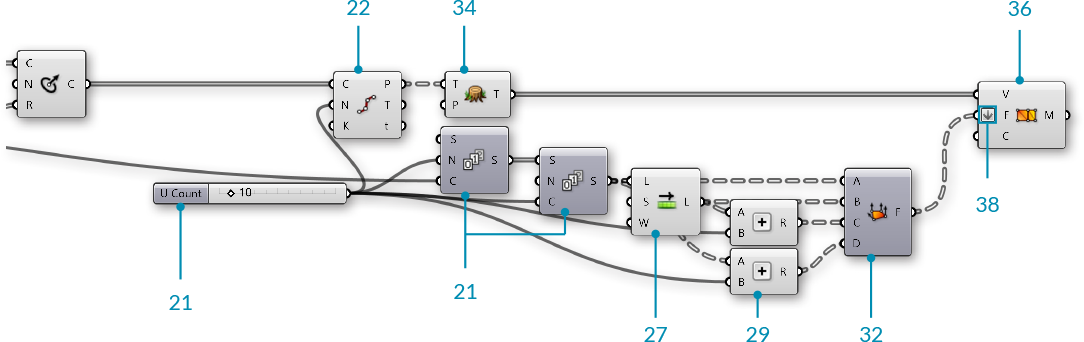We now have a mesh surface for our vase.Next we will close the bottom of the vase. To do this, we will add the original origin point to our list of vertices, and then construct triangle mesh faces from the bottom edge to that point.

39. Sets/Sequence/Series - Drag and drop a Series component onto the canvas
40. Connect the U Count number slider to the Count (C) input of the Series component
41. Sets/List/List Length - Drag and drop a List Length component onto the canvas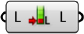42. Connect the Tree (T) output of the Flatten Tree component to the List (L) input of the List Length component

This will be the index of the origin point after we add it to the existing list of vertices.
43. Sets/List/Shift List - Drag and drop a Shift List component onto the canvas44. Mesh/Primitive/Mesh Triangle - Drag and drop a Mesh Triangle component onto the canvas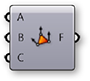45. Connect the following to the inputs of the Mesh Triangle component:
B - **List Length**
C - **Shift List**
46. Sets/Tree/Merge - Drag and drop two Merge components onto the canvas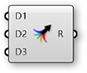47. Connect the Tree (T) output of the Flatten Tree component to the D1 input, and connect the initial Point component to the D2 input of the first Merge component
48. Connect the Faces (F) of the Mesh Quad component to the D1 input, and connect the Mesh Triangle output to the D2 input of the second Merge component
49. Connect the first Merge component to the Vertices (V) input of the Construct Mesh component, and connect the second Merge component to the Faces (F) input of the Construct Mesh component.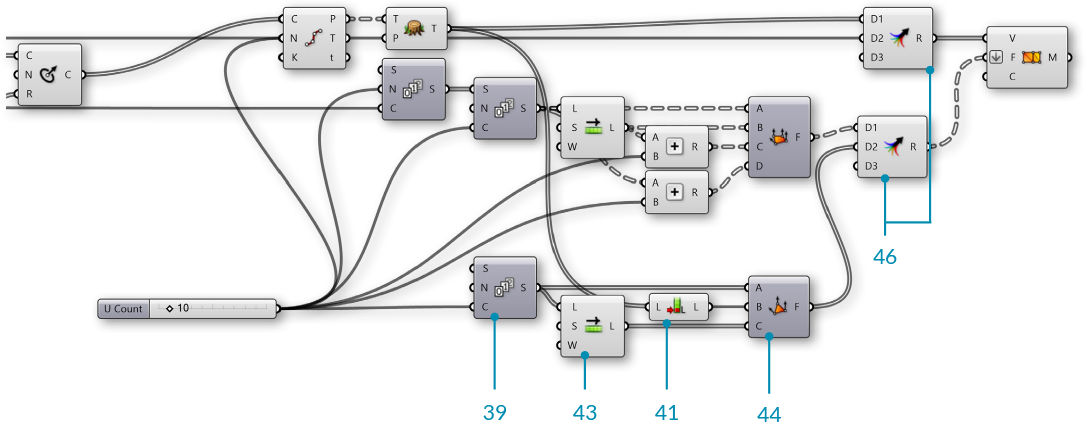We have capped the bottom of the vase with triangle mesh faces.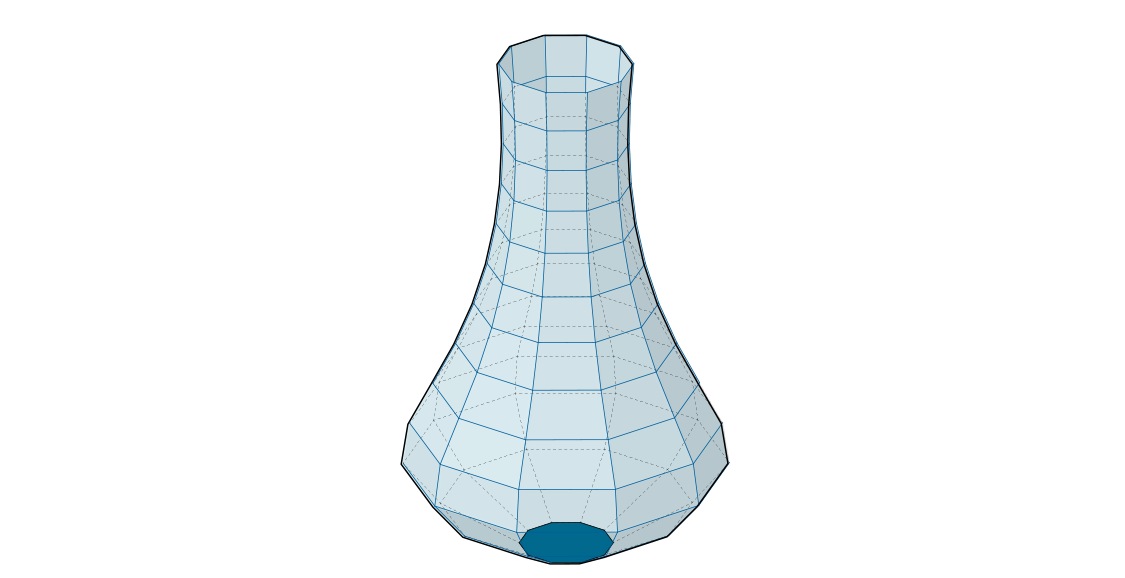We will now add some detailing to the vase. We will start by adding a curve to the vertical direction by adjusting the seam of the original circles

50. Curve/Util/Seam - Drag and drop a Seam component onto the canvas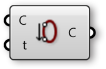51. Connect the Circle (C) output of the Circle CNR component to the Curve (C) input of the Seam component
52. Right click the Curve (C) input of the Seam component and select 'Reparameterize'
53. Params/Input/Number Slider - Drag and drop a Number Slider component onto the canvas. We will use the default settings for this slider
54. Maths/Operator/Multiplication - Drag and drop a Multiplication component onto the canvas.55. Connect the output from the Graph Mapper to the A input, and the newest Number Slider to the B input of the Multiplication component
56. Connect the Result (R) of the Multiplication component to the Parameter (t) input of the Seam component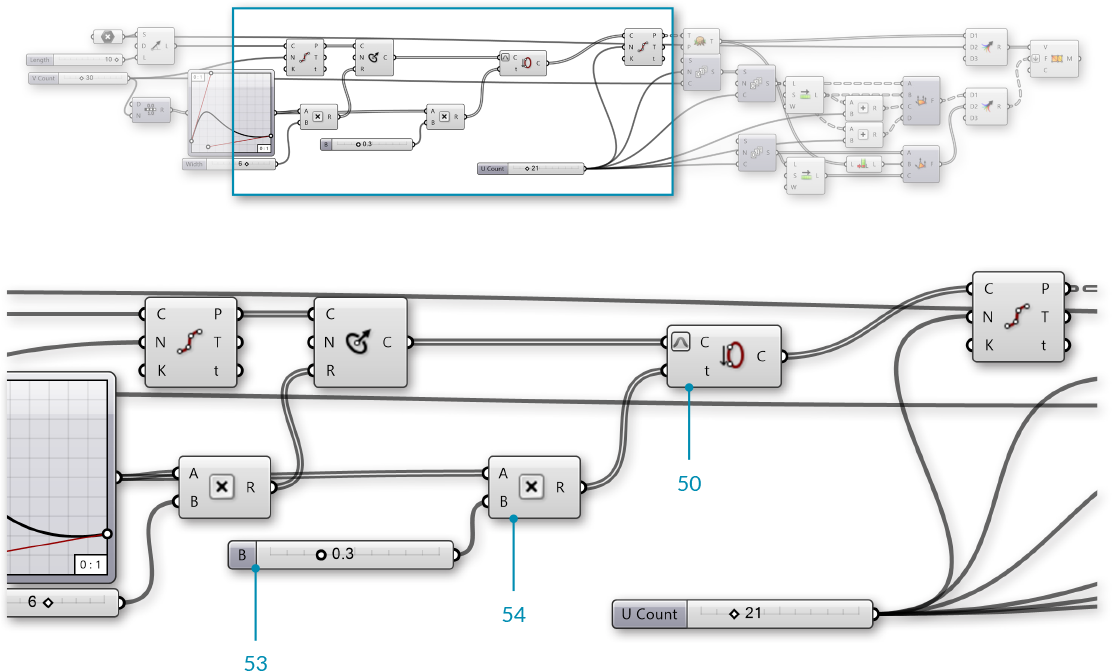The curvature is achieved by changing the seam position of the initial circles, and uses the same Graph Mapper as the vase profile.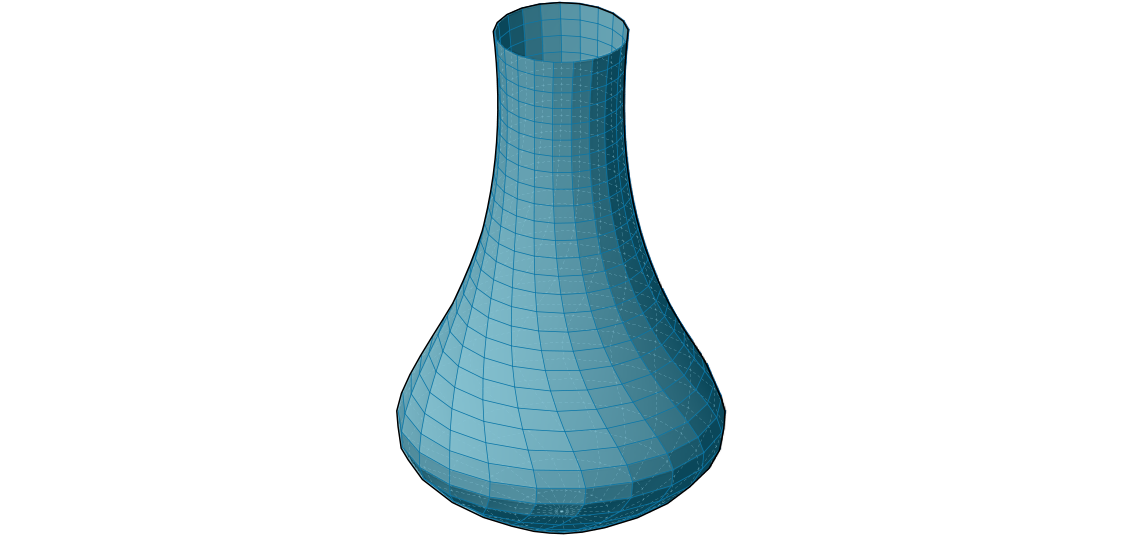Next we will add some vertical ridges to the vase.

57. Sets/List/Dispatch - Drag and drop a Dispatch component onto the canvas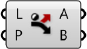58. Connect the Point (P) output of the second Divide Curve component to the List (L) input of the Dispatch component

We are using the default Pattern (P) input of the **Dispatch** component to separate the points into two lists with alternating points
59. Vector/Vector/Vector 2Pt - Drag and drop a Vector 2Pt component onto the canvas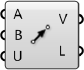60. Connect the B output of the Dispatch component to the A input of the Vector 2Pt component
61. Connect the Points (P) of the first Divide Curve component to the B input of the Vector 2Pt component
62. Right-click the B input of the Vector 2Pt component and select 'Graft', and right-click the Unitize (U) input, go to 'Set Boolean' and select 'True'

This creates a unit vector for each point that points towards the center of the circle
63. Params/Input/Number Slider - Drag and drop a Number Slider component onto the canvas. We will use the default settings
64. Maths/Operator/Multiplication - Drag and drop a Multiplication component onto the canvas65. Connect the Vector (V) output of the Vector 2Pt component to the A input, and connect the Number Slider to the B input of the Multiplication component
66. Transform/Euclidean/Move - Drag and drop a Move component onto the canvas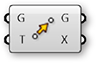67. Connect the B output of the Dispatch component to the Geometry (G) input of the Move component
68. Connect the Result (R) output of the Multiplication component to the Motion (T) input of the Move component
69. Sets/List/Weave - Drag and drop a Weave component onto the canvas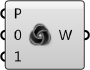70. Connect the A output of the Dispatch component to the 0 input of the Weave component
71. Connect the Geometry (G) output of the Move component to the 1 input of the Weave component
72. Connect the Weave (W) output of the Weave component to the Tree (T) input of the Flatten Tree componentRemember to go back and adjust your sliders and graph mapper to see how the model changes, and to make sure everything still works. This is known as 'flexing' the model, and should be done frequently to check for mistakes in the definition.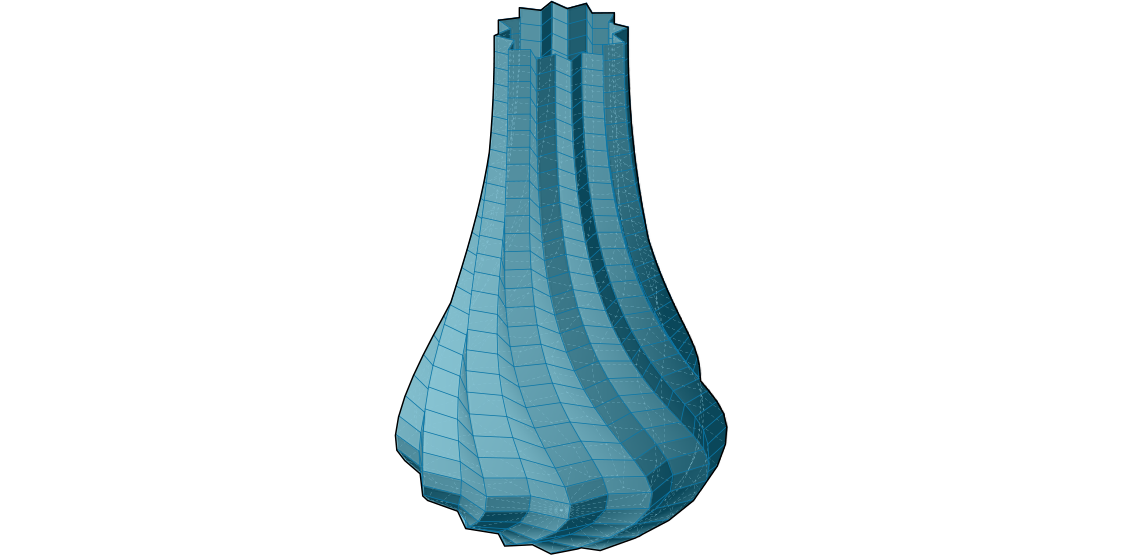We now have a single surface for our vase. If we wanted to print this vase using a 3D printer, we need it to be a closed solid. We will create a solid by offsetting the current mesh, then combining the original mesh and the offset mesh.

73. Mesh/Analysis/Deconstruct Mesh - Drag and drop a Deconstruct Mesh component onto the canvas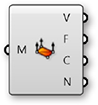74. Connect the Mesh (M) output of the Construct Mesh component to the Mesh (M) input of the Deconstruct Mesh component
75. Params/Input/Number Slider - Drag and drop a Number Slider component onto the canvas. We will use the default settings
76. Maths/Operator/Multiplication - Drag and drop a Multiplication component onto the canvas77. Connect the Normals (N) output of the Deconstruct Mesh component to the A input, and connect the Number Slider to the B input of the Multiplication component
78. Transform/Euclidean/Move - Drag and drop a Move component onto the canvas79. Connect the Vertices (V) output of the Deconstruct Mesh component to Geometry (G) input of the Move component
80. Connect the Result (R) output of the Multiplication component to the Motion (T) input of the Move component
81. Mesh/Primitive/Construct Mesh Drag and drop a Construct Mesh component onto the canvas82. Connect the Geometry (G) output of the Move component to the Vertices (V) input of the Construct Mesh component
83. Connect the Faces (F) output of the Deconstruct Mesh component to the Face (F) input of the Construct Mesh component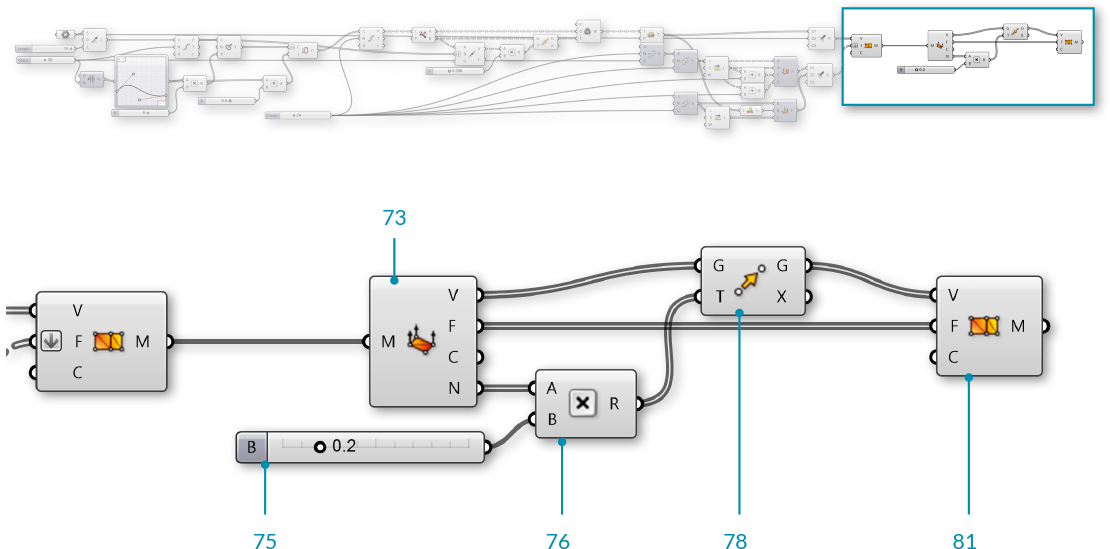By offseting the mesh according to the vertex normals, we now have an 'inside' and an 'outside' mesh, but we still have a gap at the top between the two mesh geometries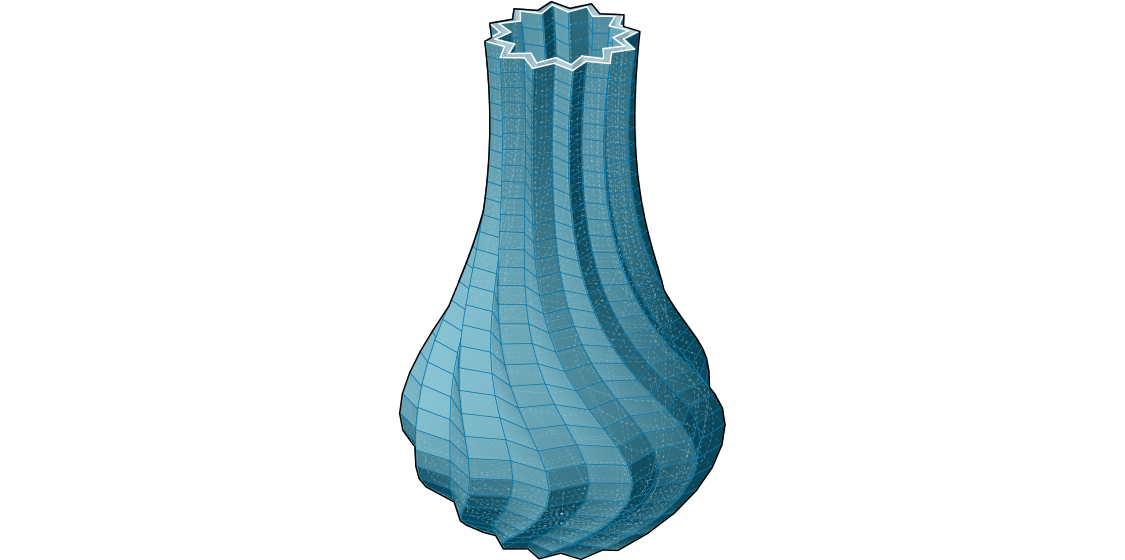The final step will be to create a closed mesh by creating a new mesh geometry to close the gap and then joining the meshes together.

84. Mesh/Analysis/Mesh Edges - Drag and drop a Mesh Edges component onto the canvas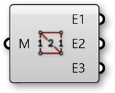85. Connect the Mesh (M) output of the first Construct Mesh component to the Mesh (M) input of the Mesh Edges component
86. Curve/Util/Join Curves - Drag and drop a Join Curves component onto the canvas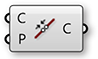87. Connect the Naked Edges (E1) output of the Mesh Edges component to the Curves (C) input of the Join Curves component
88. Curve/Analysis/Control Points - Drag and drop a Control Points component onto the canvas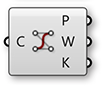89. Connect the Curves (C) output of the Join Curves component to the Curve (C) input of the Control Points component

By joining the curves and then extrating the control points, we ensure that the order of the points is consistent along the rim of the vase, which is important for making the resulting mesh orientable and manifold
90. Sets/List/Shift List - Drag and drop a Shift List component onto the canvas91. Connect the Points (P) output of the Control Points component to the List (L) input of the Shift List component
92. Repeat steps 84 through 91 for the second Construct Mesh component
93. Sets/Tree/Entwine - Drag and drop an Entwine component onto the canvas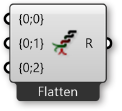94. Zoom in to the Entwine component to show the option to add an extra input. We will need four inputs. Connect them in the following way:
{0;0} - Points (P) from first **Control Points** component
{0;1} - output from first **Shift List**
{0;2} - output from second **Shift List**
{0;3} - Points (P) from second **Control Points** component
95. Sets/Tree/Flip Matrix - Drag and drop a Flip Matrix component onto the canvas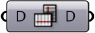96. Connect the Result (R) from the Entwine component to the Data (D) input of the Flip Matrix component
97. Mesh/Primitive/Construct Mesh - Drag and drop a Construct Mesh component onto the canvas98. Connect the Data (D) outut of the Flip Matrix component to the Vertices (V) input of the Construct Mesh component
99. Mesh/Util/Mesh Join - Drag and drop a Mesh Join component onto the canvas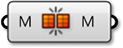100. Connect all three Construct Mesh components to the Mesh Join component by holding down the Shift key while connecting the wires (or use a Merge component). Right-click the Mesh (M) input of the Mesh Join component and select 'Flatten'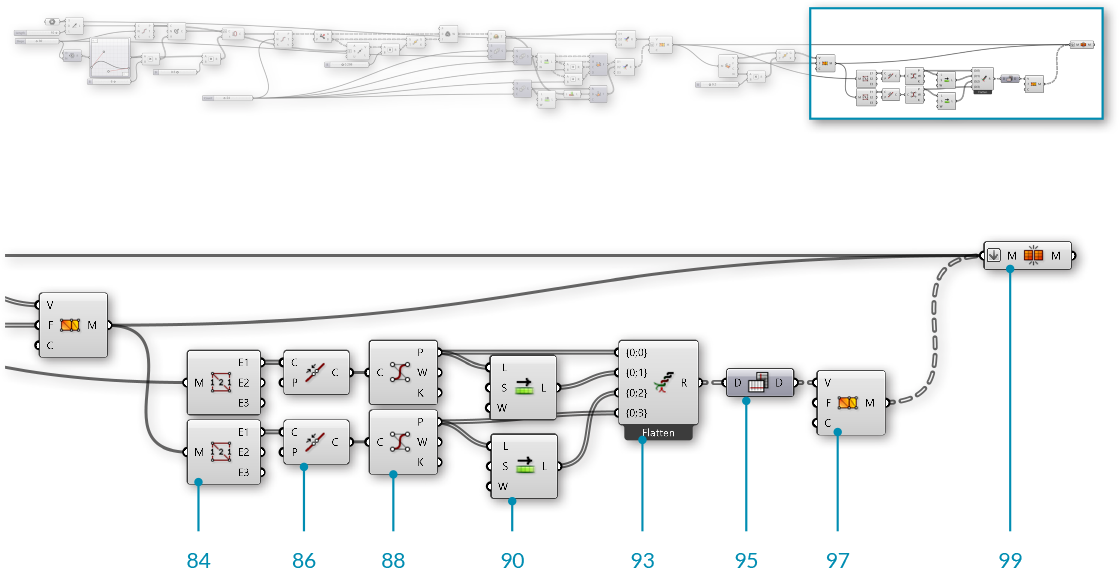##